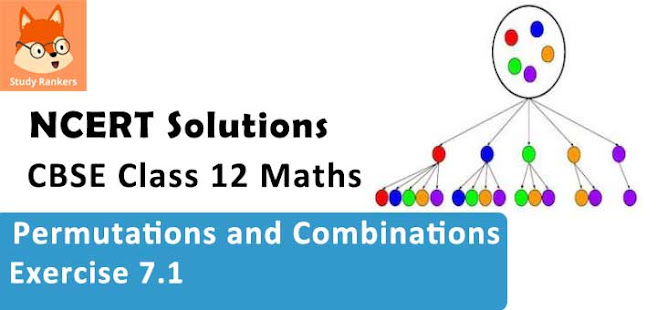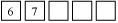# Class 11 Maths NCERT Solutions for Chapter 7 Permutations and Combinations Exercise 7.1### Permutations and Combinations Exercise 7.1 Solutions

1. How many 3-digit numbers can be formed from the digits 1, 2, 3, 4 and 5 assuming that
(i) repetition of the digits is allowed?
(ii) repetition of the digits is not allowed ?

Solution

(i) There will be as many ways as there are ways of filling 3 vacant places ⬜⬜⬜ in succession by the given five digits. In this case, repetition of digits is allowed. Therefore, the units place can be filled in by any of the given five digits. Similarly, tens and hundreds digits can be filled in by any of the given five digits.
Thus, by the multiplication principle, the number of ways in which three-digit numbers can be formed from the given digits is 5 × 5 × 5 = 125

(ii) In this case, repetition of digits is not allowed. Here, if units place is filled in first, then it can be filled by any of the given five digits. Therefore, the number of ways of filling the units place of the three-digit number is 5.
Then, the tens place can be filled with any of the remaining four digits and the hundreds place can be filled with any of the remaining three digits.
Thus, by the multiplication principle, the number of ways in which three-digit numbers can be formed without repeating the given digits is 5 × 4 × 3 = 60

2. How many 3-digit even numbers can be formed from the digits 1, 2, 3, 4, 5, 6 if the digits can be repeated?

Solution

There will be as many ways as there are ways of filling 3 vacant places ⬜⬜⬜ in succession by the given six digits. In this case, the units place can be filled by 2 or 4 or 6 only i.e., the units place can be filled in 3 ways. The tens place can be filled by any of the 6 digits in 6 different ways and also the hundreds place can be filled by any of the 6 digits in 6 different ways, as the digits can be repeated.
Therefore, by multiplication principle, the required number of three digit even numbers is 3 × 6 × 6 = 108.

3. How many 4-letter code can be formed using the first 10 letters of the English alphabet, if no letter can be repeated?

Solution

There are as many codes as there are ways of filling 4 vacant places ⬜⬜⬜⬜ in succession by the first 10 letters of the English alphabet, keeping in mind that the repetition of letters is not allowed.
The first place can be filled in 10 different ways by any of the first 10 letters of the English alphabet following which, the second place can be filled in by any of the remaining letters in 9 different ways. The third place can be filled in by any of the remaining 8 letters in 8 different ways and the fourth place can be filled in by any of the remaining 7 letters in 7 different ways.
Therefore, by multiplication principle, the required numbers of ways in which 4 vacant places can be filled is 10 × 9 × 8 × 7 = 5040
Hence, 5040 four-letter codes can be formed using the first 10 letters of the English alphabet, if no letter is repeated.

4. How many 5–digit telephone numbers can be constructed using the digits 0 to 9 if each number starts with 67 and no digit appears more than once?

Solution

It is given that the 5-digit telephone numbers always start with 67.
Therefore, there will be as many phone numbers as there are ways of filling 3 vacantplaces by the digits 0 – 9, keeping in mind that the digits cannot be repeated.
The units place can be filled by any of the digits from 0 – 9, except digits 6 and 7. Therefore, the units place can be filled in 8 different ways following which, the tens place can be filled in by any of the remaining 7 digits in 7 different ways, and the hundreds place can be filled in by any of the remaining 6 digits in 6 different ways.
Therefore, by multiplication principle, the required number of ways in which 5-digit telephone numbers can be constructed is 8 × 7 × 6 = 336

5. A coin is tossed 3 times and the outcomes are recorded. How many possible outcomes are there?

Solution

When a coin is tossed once, the number of outcomes is 2 (Head and tail) i.e., in each throw, the number of ways of showing a different face is 2.
Thus, by multiplication principle, the required number of possible outcomes is 2 × 2 × 2 = 8

6. Given 5 flags of different colours, how many different signals can be generated if each signal requires the use of 2 flags, one below the other?

Solution

Each signal requires the use of 2 flags.
There will be as many flags as there are ways of filling in 2 vacant places ⊟ in succession by the given 5 flags of different colours.
The upper vacant place can be filled in 5 different ways by any one of the 5 flags following which, the lower vacant place can be filled in 4 different ways by any one of the remaining 4 different flags.
Thus, by multiplication principle, the number of different signals that can be generated is 5 × 4 = 20